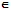# Define whole numbers in algebra

Publish On: 2019-03-16

Total Post: 559

# Mjay Jollay

Total Post: 0

## ANS: Define whole numbers in algebra

We have seen that in the set of natural number N, there exists an element 1N such that 1.m = m.1 = m, ∀ mN and that there does not exist any number (say) 0N such that

m + 0 = 0 + m = m,                                          (1)

So we extend N to a system W which we define as

W = {0, 1, 2, 3, …….}.

Where 0W satisfies

0 + m = m + 0 = m            ∀ mW.                   (2)

It is easy to say that properties, which are satisfied by N, are also satisfied by W along with the property (1), except that in the multiplicative cancellation law, where p > 0.

Also 0W has the property that

0.m = m.0 = 0              ∀ mW.